Case Based Questions (MCQ)

Chapter 8 Class 10 Introduction to Trignometry
Serial order wise

## Authority wants to construct a slide in a city park  for children. The slide was to be constructed for  children below the age of 12 years. Authority  prefers the top of the slide at a height of 4 m above  the ground and inclined at an angle of 30° to the  ground.  Based on the following figure related to the slide  answer the questions: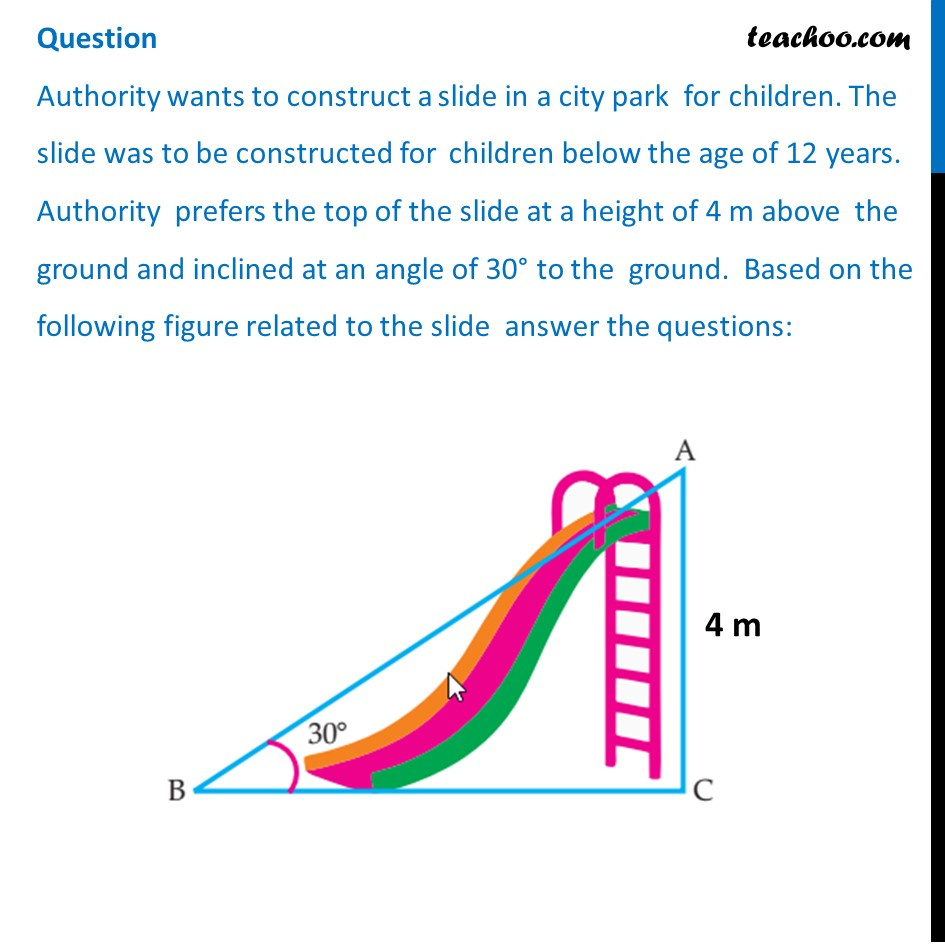## (c) 5 m          (d) 10 m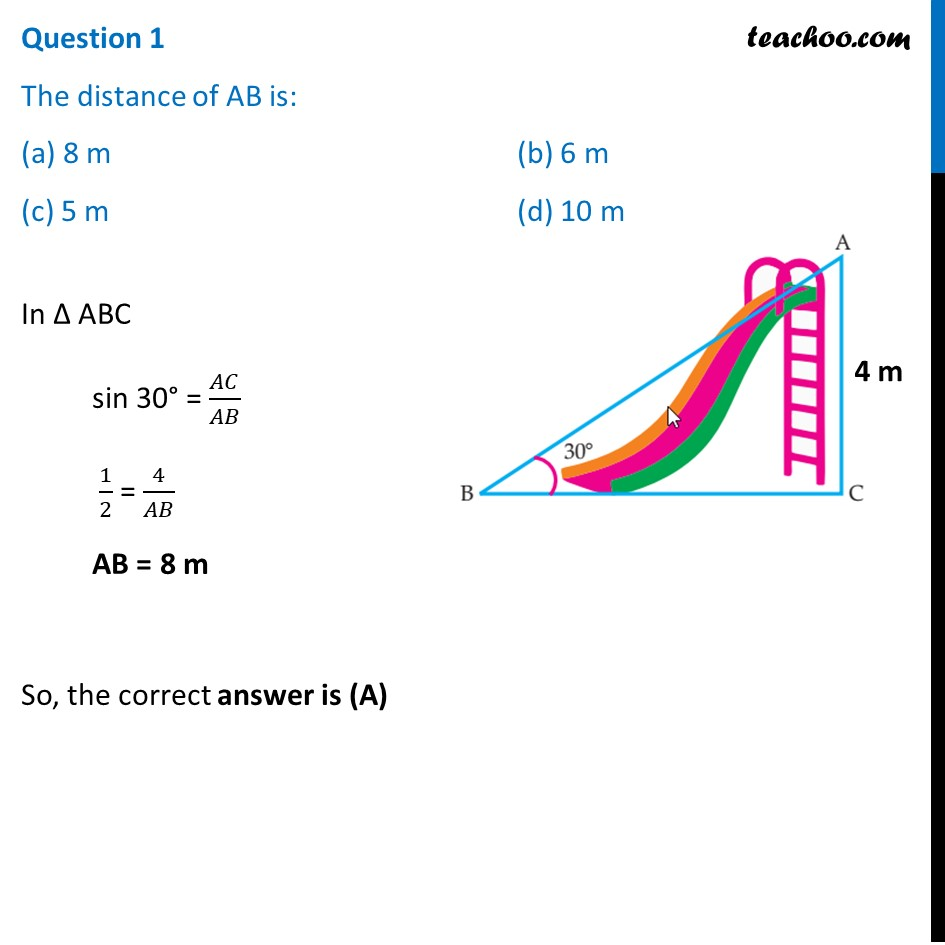## (c) 3/4                            (d) 3/2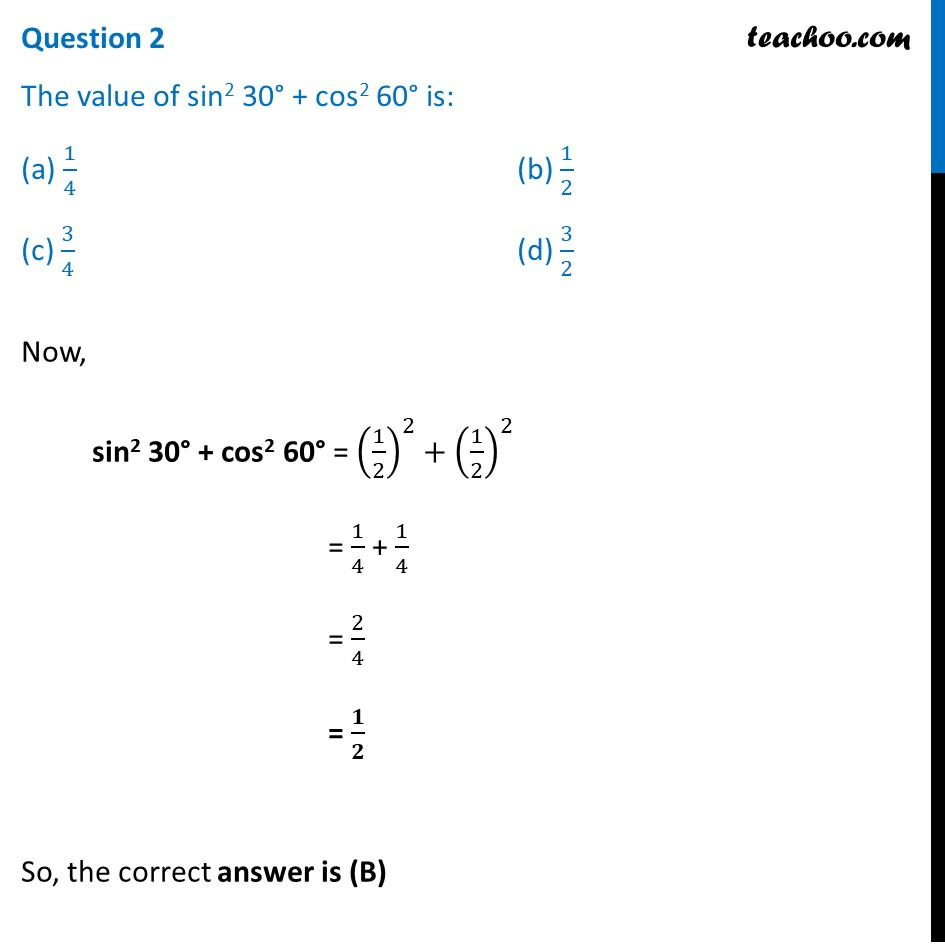## (c) 3                                                  (d) 2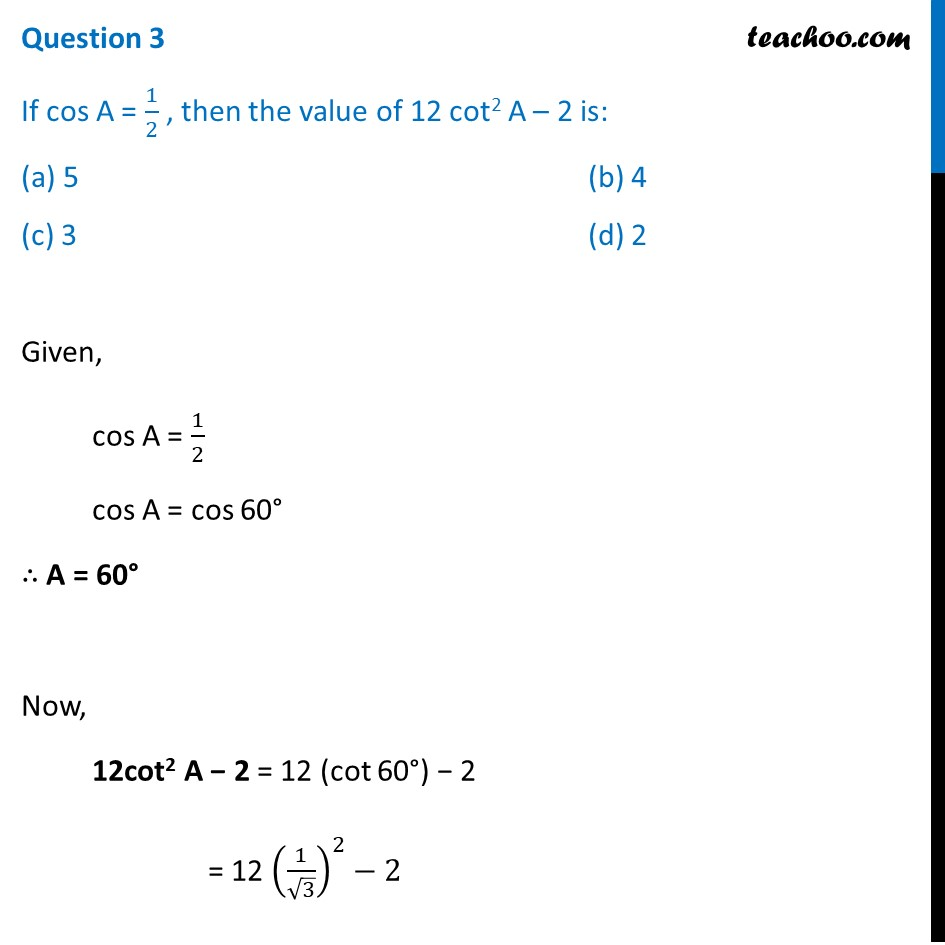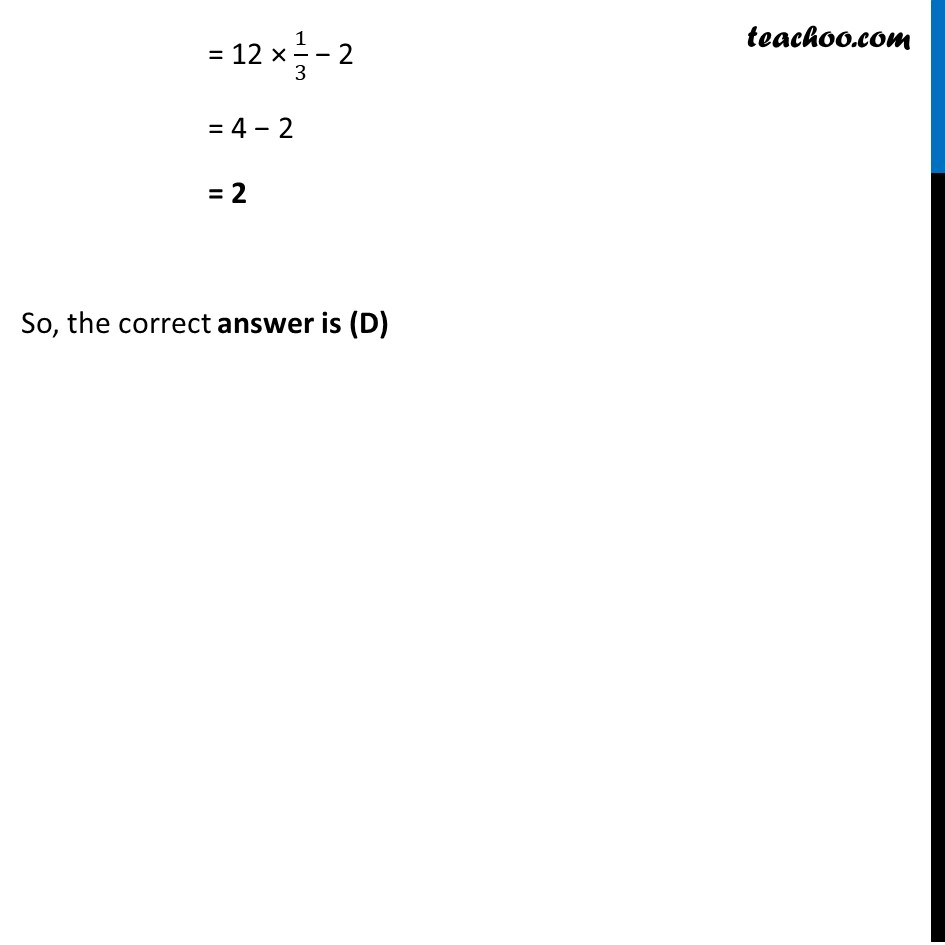## (c) 1/4                                 (d) 1/5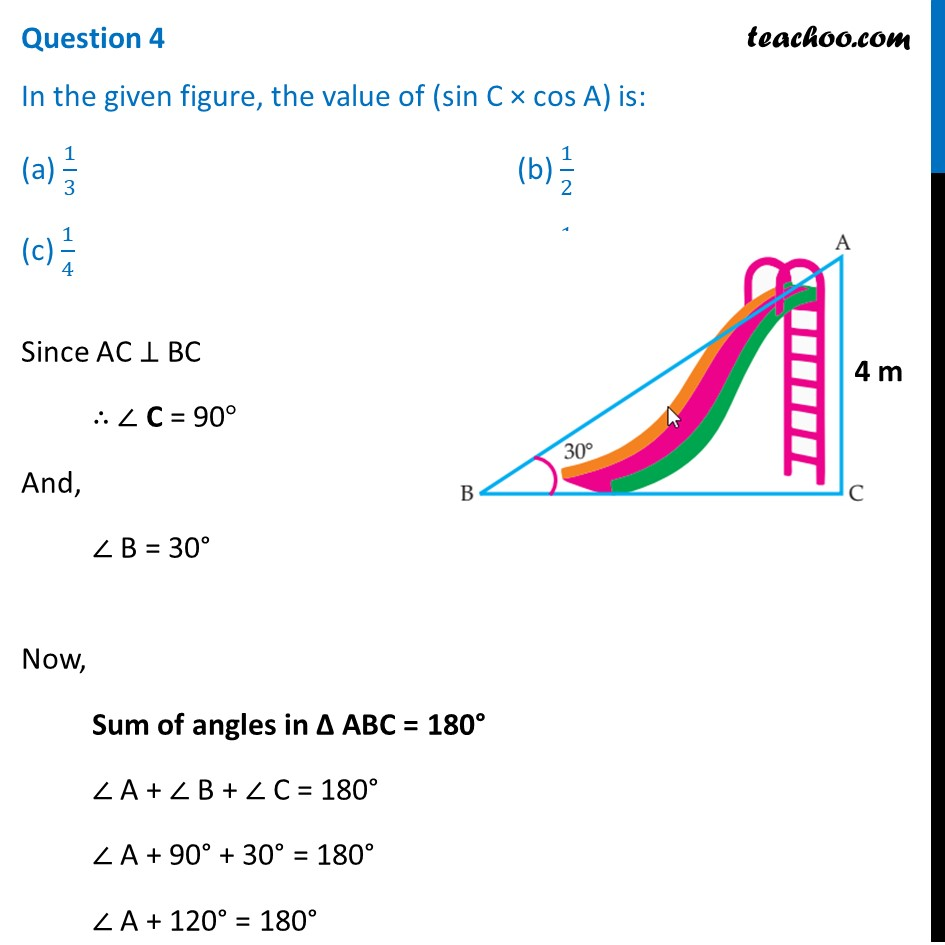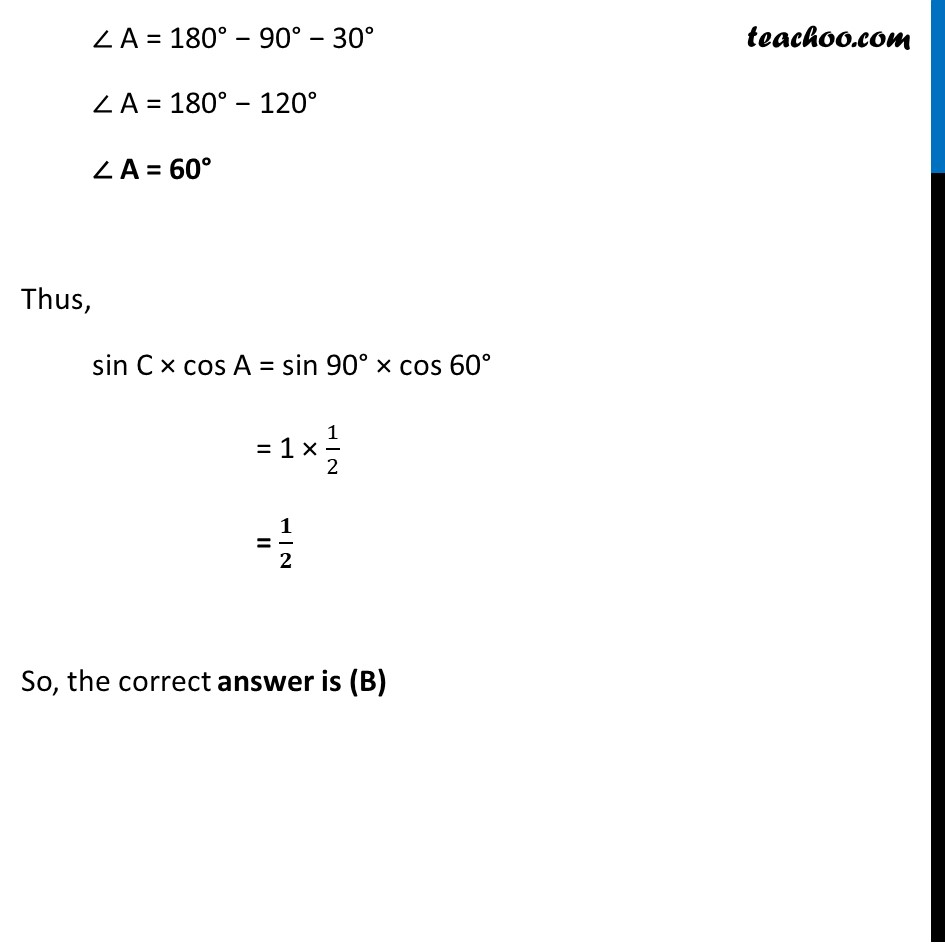## (c) 10 cm                                                        (d) 12 cm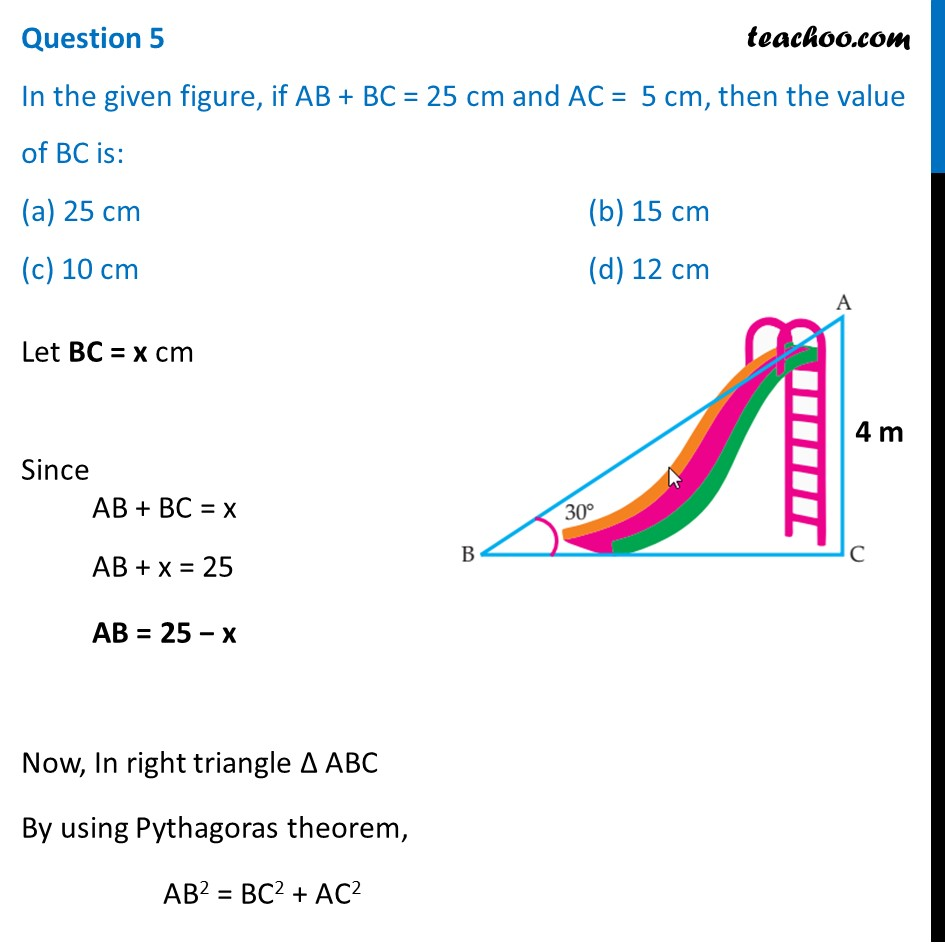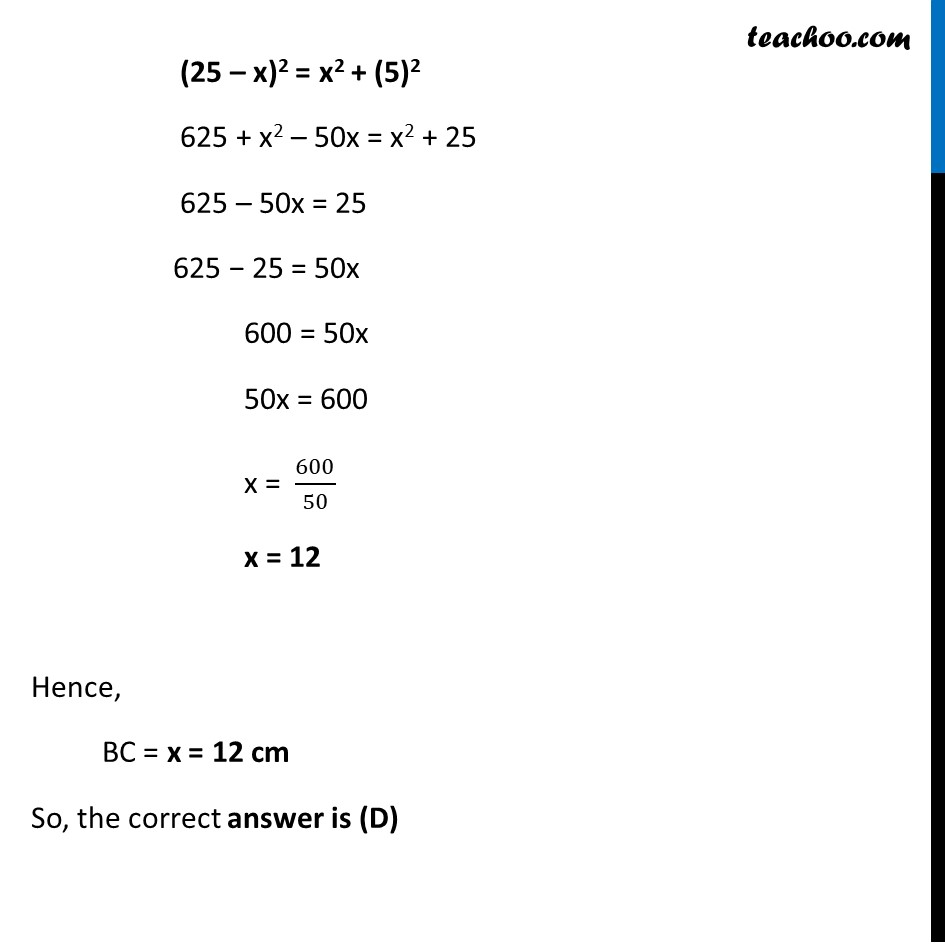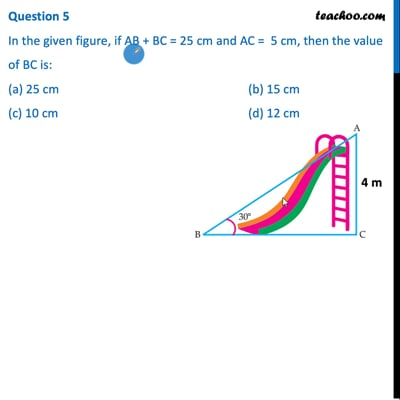This video is only available for Teachoo black users

Introducing your new favourite teacher - Teachoo Black, at only ₹83 per month

### Transcript

Question Authority wants to construct a slide in a city park for children. The slide was to be constructed for children below the age of 12 years. Authority prefers the top of the slide at a height of 4 m above the ground and inclined at an angle of 30° to the ground. Based on the following figure related to the slide answer the questions: Question 1 The distance of AB is: (a) 8 m (b) 6 m (c) 5 m (d) 10 m In Δ ABC sin 30° = 𝐴𝐶/𝐴𝐵 1/2 = 4/𝐴𝐵 AB = 8 m So, the correct answer is (A) Question 2 The value of sin2 30° + cos2 60° is: (a) 1/4 (b) 1/2 (c) 3/4 (d) 3/2 Now, sin2 30° + cos2 60° = (1/2)^2+(1/2)^2 = 1/4 + 1/4 = 2/4 = 𝟏/𝟐 So, the correct answer is (B) Question 3 If cos A = 1/2 , then the value of 12 cot2 A – 2 is: (a) 5 (b) 4 (c) 3 (d) 2 Given, cos A = 1/2 cos A = cos 60° ∴ A = 60° Now, 12cot2 A − 2 = 12 (cot 60°) − 2 = 12 (1/√3)^2−2 = 12 × 1/3 − 2 = 4 − 2 = 2 So, the correct answer is (D) Question 4 In the given figure, the value of (sin C × cos A) is: (a) 1/3 (b) 1/2 (c) 1/4 (d) 1/5 Since AC ⊥ BC ∴ ∠ C = 90° And, ∠ B = 30° Now, Sum of angles in Δ ABC = 180° ∠ A + ∠ B + ∠ C = 180° ∠ A + 90° + 30° = 180° ∠ A + 120° = 180° ∠ A = 180° − 90° − 30° ∠ A = 180° − 120° ∠ A = 60° Thus, sin C × cos A = sin 90° × cos 60° = 1 × 1/2 = 𝟏/𝟐 So, the correct answer is (B) Question 5 In the given figure, if AB + BC = 25 cm and AC = 5 cm, then the value of BC is: (a) 25 cm (b) 15 cm (c) 10 cm (d) 12 cm Let BC = x cm Since AB + BC = x AB + x = 25 AB = 25 − x Now, In right triangle Δ ABC By using Pythagoras theorem, AB2 = BC2 + AC2 (25 – x)2 = x2 + (5)2 625 + x2 – 50x = x2 + 25 625 – 50x = 25 625 − 25 = 50x 600 = 50x 50x = 600 x = 600/50 x = 12 Hence, BC = x = 12 cm So, the correct answer is (D)